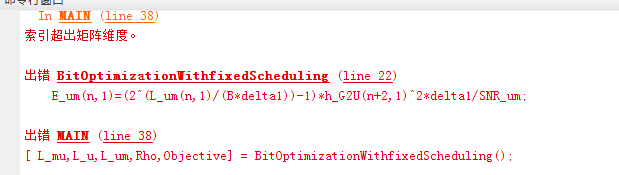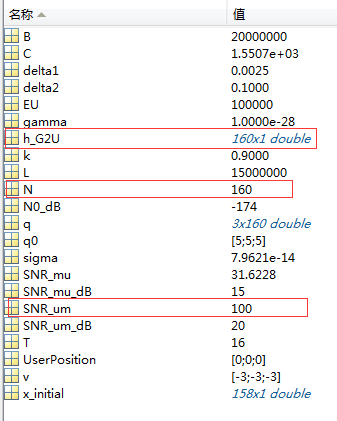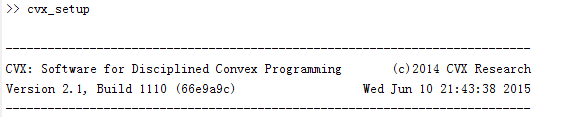# The index is beyond the matrix dimension

Dear everyone,

I encounter one problem in CVX. The matlab code is provided below.
function [ L_mu,L_u,L_um,Rho,Objective] = BitOptimizationWithfixedScheduling()

``````global h_G2U  EU k L N SNR_um SNR_mu C gamma T B delta1
cvx_begin
cvx_solver mosek
variable  Rho
variable  L_mu(N-2,1) nonnegative
variable  L_um(N-2,1) nonnegative
variable  L_u(N-2,1) nonnegative
expression  E_mu(N-2,1)
expression  E_m
expression  E_u(N-2,1)
expression  E_um(N-2,1)
%%
for n=1:N-2
E_um(n,1)=(2^(L_um(n,1)/(B*delta1))-1)*h_G2U(n+2,1)^2*delta1/SNR_um;
end
for n=1:N-2
E_u(n,1)=ComputEnergy(L_u(n,1));
end
%%

E_m=C^3*gamma*pow_pos(Rho*L,3)./T^2;

for n=1:N-2
E_mu(n,1)=CommunEnergy(L_mu(n,1), h_G2U(n,1),SNR_mu);
end
% %%
minimize sum(E_mu)+E_m
subject to
sum(E_um)+sum(E_u)<=EU;
for n=1:N-2
sum(L_u(1:n,1))<=sum(L_mu(1:n,1));
end
for n=1:N-2
sum(L_um(1:n,1))<=k*sum(L_u(1:n,1));
end
sum(L_mu)==(1-Rho)*L;
sum(L_u)==(1-Rho)*L;
sum(L_um)==k*(1-Rho)*L;
0<=Rho<=1;
cvx_end
Objective=cvx_optval;
end
``````

%%%%%%%%%%%%%%%%%%%%%%%%
Maltab window shows CVX index exceeds matrix dimensions.I have carefully checked the matrix dimension one by one, and find the dimensions of matrices are well matched. what’s wrong with it ?
The other parameters are scalar variables, which is given as followingHope someone can help me, thanks in advance.

Are you using CVX 30.beta? If so, try CVX 2.1.

Have you done `whos` immediately before the for loop in which the error occurs?

Dear Mark, these results are ran in CVX 2.1 , not CVX3.0beta.The parameters like `B, delta1, h_G2U, SNR_um` are all constant scalars or vectors. And are given as following. I type `whos` before for loop.

``````Name                Size             Bytes  Class     Attributes

B                   1x1                  8  double    global
C                   1x1                  8  double    global
EU                  1x1                  8  double    global
L                   1x1                  8  double    global
N                   1x1                  8  double    global
N0_dB               1x1                  8  double
SNR_mu              1x1                  8  double    global
SNR_mu_dB           1x1                  8  double
SNR_um              1x1                  8  double    global
SNR_um_dB           1x1                  8  double
T                   1x1                  8  double    global
UserPosition        3x1                 24  double
ans                 0x0               1281  cvx
delta1              1x1                  8  double    global
delta2              1x1                  8  double    global
gamma               1x1                  8  double    global
h_G2U             160x1               1280  double
k                   1x1                  8  double    global
q                   3x160             3840  double
q0                  3x1                 24  double
sigma               1x1                  8  double    global
v                   3x1                 24  double``````

is `whos` immediately before the for loop? If so, all of your declared CVX variables and expressions should be shown, and listed as Class `cvx` But none of them are shown.

Edit: Let’s see the results of a properly executed `whos`. Either you made a mistake or you have encountered a CVX bug. CVX_SDP Index exceeds matrix dimensions problem

Dear Mark,

I have found the mistake. The global parameter `h_G2U` miss stated in function. Thank you for your suggestions.

global variables are very dangerous. It is easy for bad things to happen when you use them without sufficient care.

Thank you for your suggestion. It help me a lot.